## Gaussian Function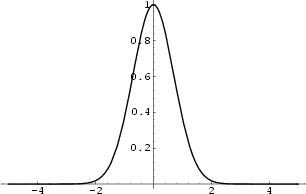In 1-D, the Gaussian function is the function from the Gaussian Distribution,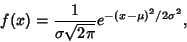(1)

sometimes also called the Frequency Curve. The Full Width at Half Maximum (FWHM) for a Gaussian is found by finding the half-maximum points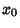. The constant scaling factor can be ignored, so we must solve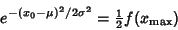(2)

But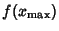occurs at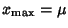, so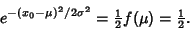(3)

Solving,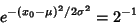(4)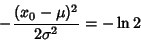(5)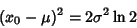(6)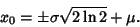(7)

The Full Width at Half Maximum is therefore given by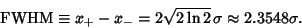(8)

In 2-D, the circular Gaussian function is the distribution function for uncorrelated variablesandhaving a Gaussian Bivariate Distribution and equal Standard Deviation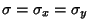,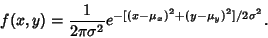(9)

The corresponding elliptical Gaussian function corresponding to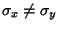is given by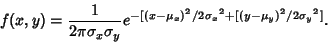(10)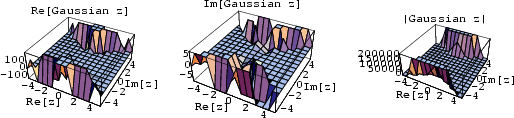The above plots show the real and imaginary parts of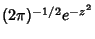together with the complex absolute value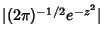.The Gaussian function can also be used as an Apodization Function, shown above with the corresponding Instrument Function.

The Hypergeometric Function is also sometimes known as the Gaussian function.

See also Erf, Erfc, Fourier Transform--Gaussian, Gaussian Bivariate Distribution, Gaussian Distribution, Normal Distribution

References

MacTutor History of Mathematics Archive. Frequency Curve.'' http://www-groups.dcs.st-and.ac.uk/~history/Curves/Frequency.html.